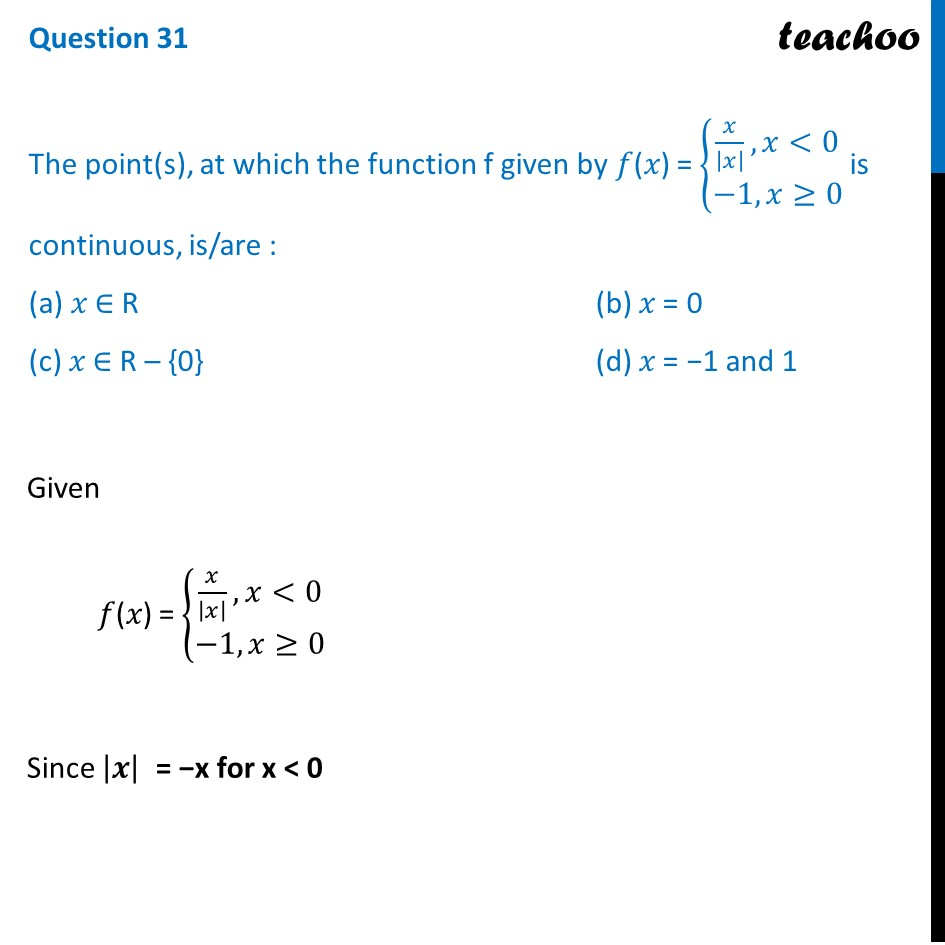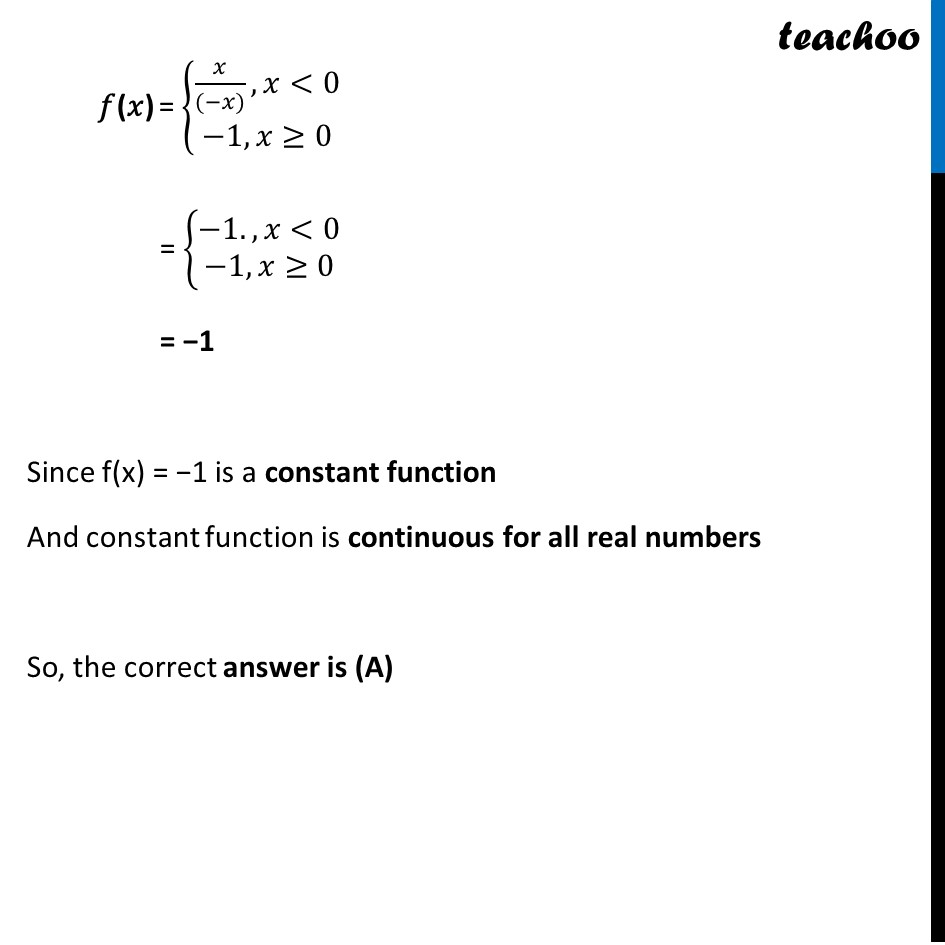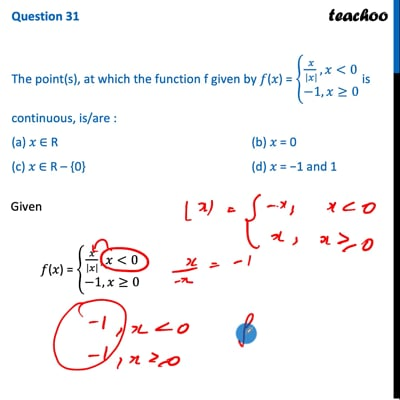CBSE Class 12 Sample Paper for 2022 Boards (MCQ Based - for Term 1)

Class 12
Solutions of Sample Papers and Past Year Papers - for Class 12 Boards

## (c) 𝑥 ∈ R – {0}         (d) 𝑥 = −1 and 1This video is only available for Teachoo black users

Get live Maths 1-on-1 Classs - Class 6 to 12

### Transcript

Question 31 The point(s), at which the function f given by 𝑓(𝑥) = {■8(𝑥/|𝑥| ,𝑥<[email protected]−1, 𝑥≥0)┤ is continuous, is/are : (a) 𝑥 ∈ R (b) 𝑥 = 0 (c) 𝑥 ∈ R – {0} (d) 𝑥 = −1 and 1 Given 𝑓(𝑥) = {■8(𝑥/|𝑥| ,𝑥<[email protected]−1, 𝑥≥0)┤ Since |𝒙| = −x for x < 0 𝑓(𝑥) = {■8(𝑥/((−𝑥)),𝑥<[email protected]−1, 𝑥≥0)┤ = {■8(−1. ,𝑥<[email protected]−1, 𝑥≥0)┤ = −1 Since f(x) = −1 is a constant function And constant function is continuous for all real numbers So, the correct answer is (A)